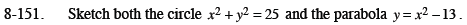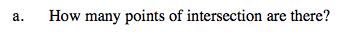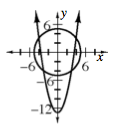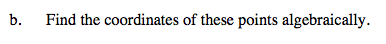Home > CCA2 > Chapter 8 > Lesson 8.3.2 > Problem8-151

8-151.
1. Sketch both the circle x2 + y2 = 25 and the parabola y = x2 − 13. Homework Help ✎

1. How many points of intersection are there?

2. Find the coordinates of these points algebraically.Look at the graph.4 pointsSubstitute x² − 13 into the first equation.

Solve for x.

Factor the equation.

Now substitute in these x values to find the y.

$x^2 + (x^2 - 13)^2 = 25$

$x^4 - 25x^2 + 144 = 0$

(x² − 16)(x² − 9)

(x − 4)(x + 4)(x − 3)(x + 3)

$x = \pm4, \pm3$